Question

A car battery has a rating of 250 ampere-hours. This rating is one indication of the total charge that the battery can provide to a circuit before failing. (a) What is the total charge (in coulombs) that this battery can provide? (b) Determine the maximum current that the battery can provide for 37 minutes.

1.Cherry

Total charge provided by the battery could be 900000 C.

Maximum current provided by the battery for 37 minutes could be 405.405 A

Explanation:

Rating= 250 A-h

a. Total charge: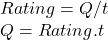Suppose t=1h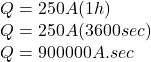We konw that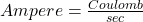, replacing: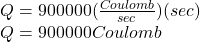Total charge provided by the battery could be 900000 C.

b. Maximum current for 37 minutes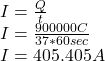Maximum current provided by the battery for 37 minutes could be 405.405 A